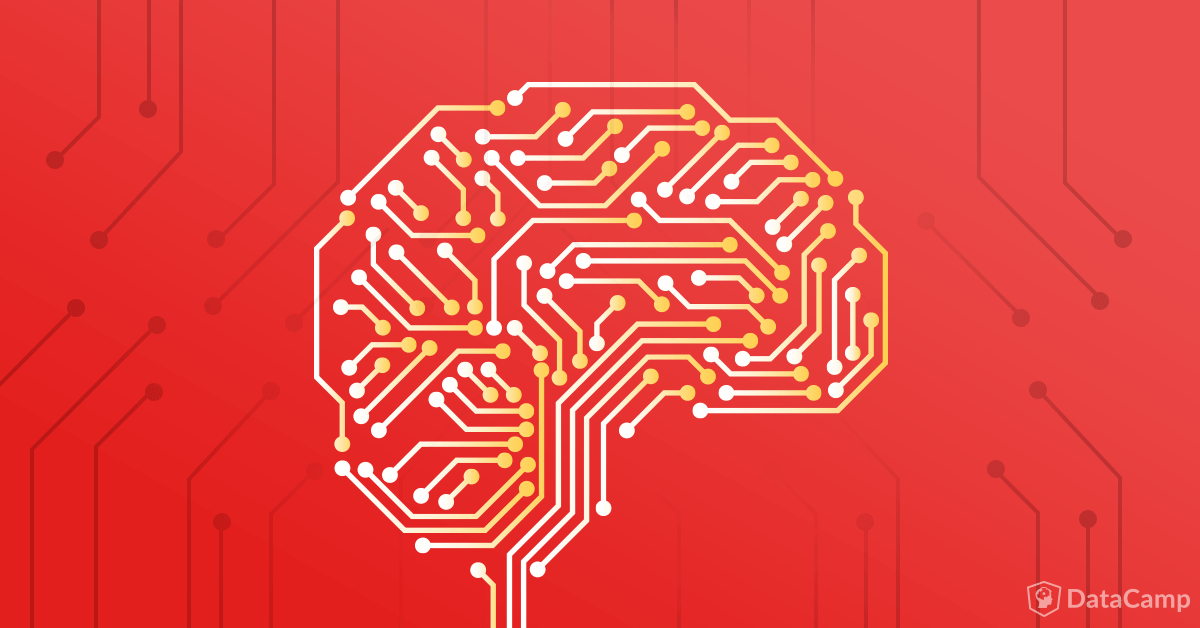# Keras Tutorial: Deep Learning in Python

This Keras tutorial introduces you to deep learning in Python: learn to preprocess your data, model, evaluate and optimize neural networks.## Deep Learning

By now, you might already know machine learning, a branch in computer science that studies the design of algorithms that can learn. Today, you’re going to focus on deep learning, a subfield of machine learning that is a set of algorithms that is inspired by the structure and function of the brain. These algorithms are usually called Artificial Neural Networks (ANN). Deep learning is one of the hottest fields in data science with many case studies that have astonishing results in robotics, image recognition and Artificial Intelligence (AI).

One of the most powerful and easy-to-use Python libraries for developing and evaluating deep learning models is Keras; It wraps the efficient numerical computation libraries Theano and TensorFlow. The advantage of this is mainly that you can get started with neural networks in an easy and fun way.Today’s Keras tutorial for beginners will introduce you to the basics of Python deep learning: